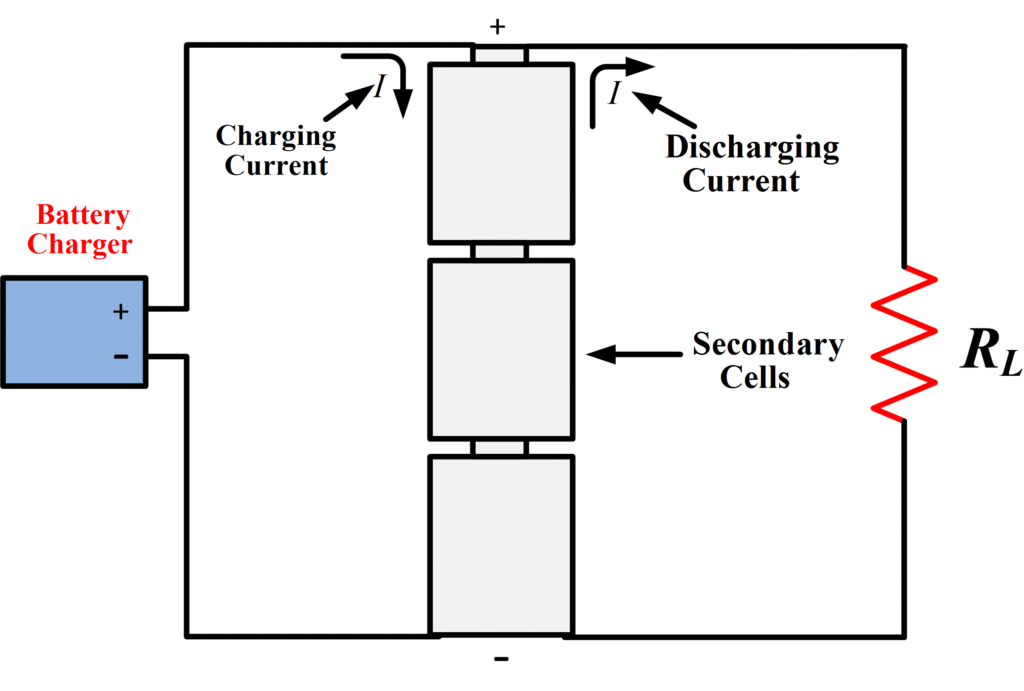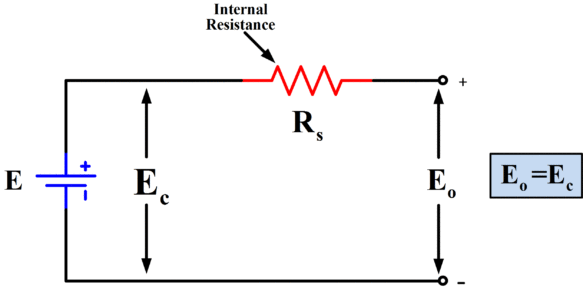# Voltaic Cell Equivalent Circuit | Voltaic Cell Example

Want create site? Find Free WordPress Themes and plugins.

## Primary and Secondary Cells

The simple voltaic cell is classiﬁed as a primary cell. Primary cells can supply current until the negative electrode is completely dissolved, the electrolyte is exhausted, or perhaps until polarization renders the cell useless. A secondary cell, on the other hand, can be recharged. That is, the electrode surface can be reformed and the electrolyte returned to its original condition. This is done by passing a current through the cell in an opposite direction to the current that normally ﬂows from the cell (see Figure 1). Most dry cells, such as the zinc-carbon cells cannot be recharged; consequently, they are primary cells.Fig.1: Current Flow in the Secondary Cells

Secondary cells are rechargeable. The charging current flows into the cell in the opposite direction to the load current.

## Voltaic Cell Equivalent Circuit

As already explained, when no current is being drawn from a voltaic cell, charges are accumulated on the electrodes, and the chemical action ceases within the electrolyte. At this time, the potential difference between the cell terminals is known as the open-circuit output voltage or the no-load output voltage.

When current ﬂows from the cell, electrons are transferred externally from the negative electrode to the positive electrode. Thus, the potential difference between the electrodes falls, and the chemical action within the electrolyte commences to replace the lost charges.

If the load current is very small, the terminal voltage under load may not be noticeably different from the open-circuit output voltage. When the load current is large, the terminal voltage falls below the open-circuit voltage level. If the load current is made too large, the cell terminal voltage falls to near zero, because the chemical action cannot occur fast enough to replace the charges removed from the electrodes. The maximum current that may be taken from a voltaic cell without a substantial drop in output voltage is proportional to the surface area of the electrodes and to the composition of the electrolyte.

To account for the terminal voltage drop of a cell when supplying current, each cell is said to have a certain internal resistance. The cell can then be represented by an equivalent circuit consisting of its internal resistance together with an ideal cell that has a terminal voltage equal to the actual cell’ s open-circuit terminal voltage. An ideal cell is a theoretical cell that has a terminal voltage that is assumed to remain constant. The equivalent circuit of a voltaic cell is illustrated in Figure 2.Fig.2 (a): Equivalent Circuit of Cell (Io=0)Fig.2 (b): Equivalent Circuit of cell supplying a load current

When a load current flows, the terminal voltage is reduced by the cell internal voltage drop.

When no current is ﬂowing from the equivalent circuit, there is no voltage drop across the internal resistance (Rs) and the terminal voltage is equal to the ideal Cell voltage. That is, the output voltage is the open circuit voltage E, of the cell [see Figure 2 (a)]. When a load resistance (RL) is connected to the cell terminals [Figure 2 (b)], the current that flows is

$\begin{matrix} {{I}_{o}}=\frac{{{E}_{c}}}{{{R}_{s}}+{{R}_{L}}} & {} & \left( 1 \right) \\\end{matrix}$

When supplying current, a voltage drop occurs across Rs, and the output voltage becomes

$\begin{matrix} {{E}_{o}}={{E}_{c}}-{{I}_{o}}{{R}_{s}} & {} & \left( 2 \right) \\\end{matrix}$

Obviously, a cell that has a low internal resistance is capable of supplying larger current than one that has a relatively high internal resistance.

## Voltaic Cell Calculations Example

A certain voltaic cell has an open-circuit terminal voltage of Ec=2V and an internal resistance of Rs=0.1Ω. Determine the output voltage of the cell when the load current is 2A. Also, determine the cell current when the terminals are short-circuited.

Solution

When Io=2A:

From equation (2):

\begin{align} & {{E}_{o}}={{E}_{c}}-{{I}_{o}}{{R}_{s}} \\ & =2-(2*0.1) \\ & =1.8V \\\end{align}

When the cell is short-circuited:

${{R}_{L}}=0$

From equation (1):

${{I}_{o}}=\frac{{{E}_{c}}}{{{R}_{s}}+{{R}_{L}}}=\frac{2}{0.1+0}=20A$

Did you find apk for android? You can find new Free Android Games and apps.